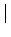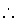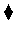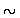Next: Trees Up: Adding Computation Domains Previous: Constructing Recursive Data Structures

Subsections

# Recursive Programming: Lists

Lists are one of the most useful data structures. They are present as primitive constructs in many languages (e.g., virtually all functional and logic languages) and available as libraries in many others. Lists can be defined by the user as any other structure in CLP languages, but they appear so often that there is a special syntax for them.

Formally, a list of elements is either the empty list (usually called nil and written []), or an element consed (put as head of'') with another list. Thus a list is always either an empty list or a head followed by a tail. This is modeled using a functor of arity 2, called cons. The name of the functor is usually .'. For example the list composed by the elements a, b and c is formally written .(a, .(b, .(c, []))). The first argument of each of the cons functor is the head of the list; the second is the tail of the list. A list term is logically defined (and recognized) by the predicate is_list/2, defined as follows:

is_list([]).


This syntax for lists reflects the logical idea, but it is not very readable nor descriptive for an intuitive use of lists. Furthermore, the dot is overloaded by its use as clause terminator, and should be written quoted. It is customary to use a combination of square brackets and the infix operator' to write lists. To make life easier, there is a special syntax for writing lists without having to separate explicitly the head(s) and tail(s). Table 3.3 shows examples of the three syntaxes.

Table 3.3: Syntaxes for lists
Formal object Cons pair syntax Element'' syntax
.(a,[ ]) [a[ ]] [a]
.(a,.(b,[ ])) [a[b[ ]]] [a,b]
.(a,.(b,.(c,[ ]))) [a[b[c[ ]]]] [a,b,c]
.(a,X) [aX] [aX]
.(a,.(b,X)) [a[bX]] [a,bX]

List matching behaves as in any other structure. Some remarks will help to understand the element syntax:

• [a,b] and [aX] unify with X = [b] (X is the tail of a list--another list itself).
• [a] and [aX] unify with X = [] (the tail of the singleton list is always the empty list).
• [a] and [a,bX] do not unify (since the first list has one element, and the second one has, at least, two).
• [] and [X] do not unify.

With this notation, the definition of lists can be expressed with the following predicate:

is_list([]).A common operation is checking for membership in a list. The member/2 predicate is true if the first argument is an element of the list which appears as second argument:

member(Element, [Element|List]).
member(Element, [AnElement|RestList]):- member(Element, RestList).


And, as in other cases, it can be used to check membership, to return on backtracking all elements which are members of a list, or to force a list to have an element as member:

?- member(b, [a, b, c]).
yes

?- member(plof, [a, b, c]).
no

?- member(X, [a, b, c]).
X = a ? ;
X = b ? ;
X = c ?

?- member(a, [a, X, c]).
true ;
X = a


Problem 3.14   What does the query member(gogo, L) return? Why?Another useful predicate is append/3: append(A, B, C) is true if C is the list constructed by concatenating the lists A and B. The definition is:

append([], X, X).
append([X|Xs], Ys, [X|Zs]):-  append(Xs, Ys, Zs).


and it can be used in multiple ways:

?- append([1, 2, 3], [g, h, t], L).
L = [1, 2, 3, g, h, t].

?- append(T, [g, h, t], [0, m, g, X, Y]).
Y = t, X = h, T = [0, m].

?- append(X, Y, [f, p, r]).
Y = [f, p, r], X = [];
Y = [p, r], X = [f];
Y = [r], X = [f, p];
Y = [], X = [f, p, r].


#### A note on Prolog IV lists

Prolog IV lists, apart from the usual behavior we have just sketched, can be constrained using special primitives: size/2, which relates a list with the number of its elements, o/3, which relates two lists with their concatenation, and index/3, which relates a list and a number with the element which is placed in the list at the position given by that number. o/3 is, in some sense, similar to append/3, but the crucial difference is that, similarly to other constraints, it does not enumerate, but instead leaves a constraint among lists. o/3 can also be written using thenotation. The examples below (specially the last one) will make this clearer:

?- Z = [1, 2, 3] o [4, 5, 6].
Z = [1, 2, 3, 4, 5, 6].

?- [1, 2, 3, 4, 5, 6] = X o [4, 5, 6].
X = [1, 2, 3].


o/3 does not enumerate, though:

?- [1, 2, 3, 4, 5, 6] = X o Y.
Y ~ list, X ~ list.


But o/3 does constrain (and size/2 does, too):

?- [1|Xs] = Xs o [\_], 4 ~ size(Xs).
Xs = [1, 1, 1, 1].


Problem 3.15   Use append/3 to make the last query. Could you explain how the answer is reached in the constraints case? Try to reason without thinking of solvers: act as a solver, and perform a step by step reasoning.We will look at another example of a useful predicate and two feasible implementations of it. Sometimes the order in a list is important (although the list could not be called ordered in the sense of the word sorted: its order may derive from other considerations, such as the order of words in a file), and a utility predicate in many cases is reverse/2, which relates a list with the result of traversing it from the last element to the first.

A first possibility is reasoning that, if we have an empty list, the empty list is its own reversed list, and, if we have a nonempty list and take apart head and tail, reverse the tail (which is a simpler problem), and append the head at the end (for which we can use the append/3 predicate), then the original list will be reversed. Putting it in code:

reverse([],[]).
reverse([X|Xs],Ys ):-
reverse(Xs,Zs),
append(Zs,[X],Ys).


This is a correct definition, but it is very inefficient: for every element in the list, the predicate has to reverse the corresponding tail, and then put that element at the end, which needs traversing the reversed list completely again. This makes this predicate to be quadratic with respect to the length of the first argument. A better strategy is using a common technique called accumulation parameter: an extra parameter is internally used, in which the final result is constructed. The original list is traversed and each element is pushed onto the argument which will be returned as final solution:

reverse(Xs, Ys):- reverse(Xs, [], Ys).

reverse([], Ys, Ys).
reverse([X|Xs], Acc, Ys):- reverse(Xs, [X|Acc], Ys).


Do not be baffled by the presence of reverse/2 and reverse/3: different arities define different predicates. reverse/3 could have been called with a completely different name, but it is just not necessary. The second argument of reverse/3 is called with an empty list, and, at every recursion step, the first element in the list to be reversed is pushed as first element of that second argument. The result is that, when recursion finishes, the second argument contains the initial list, but reversed. It is then unified with the third argument, which holds the result and which is the same variable as the result variable in the toplevel call.

Problem 3.16   What is the efficiency, in time, of this second implementation, with respect to the length of the first list?Lists are, without any doubt, the most useful data structure in CLP, and thus it is worth knowing how to use them, even if some of this knowledge might not always be necessary.

Problem 3.17   Write definitions for the following predicates (previously defined predicates may be freely used):

• len(L, N): N is the length (using Peano arithmetic) of the list L
• suffix(S, L): S is a suffix of the list L
• prefix(P, L): P is a prefix of the list L
• sublist(S, L): S is a sublist of the list L
• last(E, L): E is the last element of the list L
• palindrome(L): the list L is a palindrome
• evenodd(L, E, O): for any list L, E is the list of the elements in even position (i.e., the 2nd, 4th, etc.), and O is the list of the elements in odd position (i.e., the 1st, 3rd, etc.)
• select(E, L1, L2): L2 is the list L1 without one (any one) of its elements, E, e.g.,
?- select(X, [a,c,n], L).
L = [c ,n], X = a ;
L = [a, n], X = c ;
L = [a, c], X = n
Try to give as many solutions as you can, and pay attention to the differences in performance.In many cases keeping items ordered in a list can be advantageous, because search time can be reduced; insertion time, on the other hand, is slower, because the right place to insert an element must be found, while in an unordered list, a new list with an additional element can be constructed in constant time just by consing the new head (the element) with the tail (the previous list). We will assume that there is a generic predicate precedes/2 such that precedes(A, B) is true if A precede B in the desired order. For numeric elements, this amounts to an arithmetical comparison, but in arbitrary pieces of information it can require a more complicated implementation. The code for inserting a piece of information in an ordered list without repetitions is:

insert_ordlist(Element, [], [Element]).
insert_ordlist(Element, [This|Rest], [This, Element|Rest]):-
precedes(This, Element).
insert_ordlist(Element, [Element|Rest],  [Element|Rest]).
insert_ordlist(Element, [This|Rest], [This|NewList]):-
precedes(Element, This),
insert_ordlist(Element, Rest, NewList).


Problem 3.18   Write a variant in which repetitions are allowed.The code for searching an element in an ordered list can stop the search before going past the last item: when we find an item which should be placed after the element we are looking for, we know that the sought for term is actually not present in the list.

search_ordlist(Element, [Element|Rest]).
search_ordlist(Element, [This|Rest]):-
precedes(This, Element),
search_ordlist(Element, Rest).Next: Trees Up: Adding Computation Domains Previous: Constructing Recursive Data Structures
MCL
1998-12-03